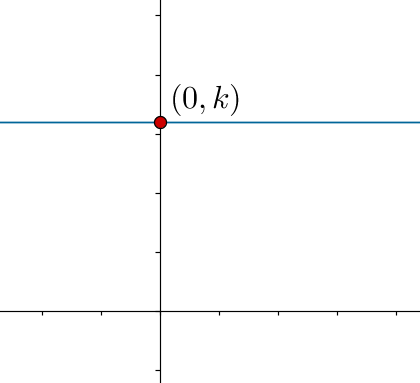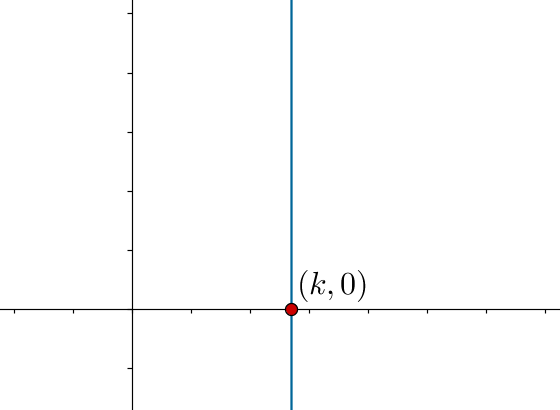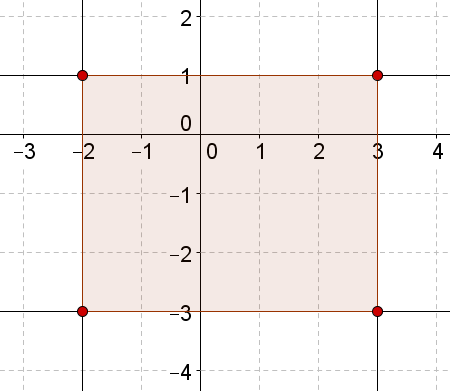In the verge of coronavirus pandemic, we are providing FREE access to our entire Online Curriculum to ensure Learning Doesn't STOP!

# Lines parallel to the Axes

Go back to  'Coordinate-Geometry'

Consider a line which is parallel to the x-axis, intersecting the y-axis at the point $$\left( {0,k} \right)$$:If $$P\left( {x,y} \right)$$ is any arbitrary point on this line, the y-coordinate of P must be equal to k (since the line is horizontal – all points have the same y-coordinate). Thus, we can say that the coordinates of P are constrained by the equation

$y = k$

In other words, the equation of the line in the figure above is $$y = k$$. Note that there is no x in the equation because y equals k regardless of the value of x.

Now, consider a line which is parallel to the y-axis, intersecting the x-axis at the point $$\left( {k,0} \right)$$ :An arbitrary point $$P\left( {x,y} \right)$$ on this line will be constrained by the equation

$x = k$

Thus, the equation of this line is $$x = k$$.

Example 1: Find the area of the rectangle formed by the lines $$x = 3$$, $$x = - 2$$, $$y = 1$$ and $$y = - 3$$.

Solution: The figure below shows the four lines, and the rectangle enclosed:<

Clearly, the area of the rectangle is $$5 \times 4 = 20$$ square units.

Coordinate Geometry
Grade 9 | Questions Set 1
Coordinate Geometry
Coordinate Geometry
Grade 10 | Questions Set 1
Coordinate Geometry